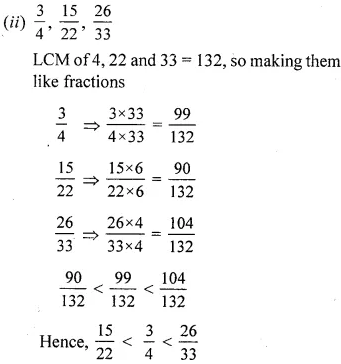# ML Aggarwal Class 6 Solutions for ICSE Maths Chapter 6 Fractions Ex 6.4

## ML Aggarwal Class 6 Solutions for ICSE Maths Chapter 6 Fractions Ex 6.4

Question 1.
Show the fractions $$\frac{1}{6}, \frac{2}{6}, \frac{3}{6}, \frac{4}{6}, \frac{5}{6}, \frac{6}{6}$$ and $$\frac{8}{6}$$ on the number line. Replace ‘……’ by an appropriate sign” between given fractions: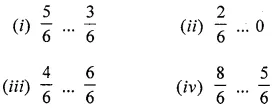Solution: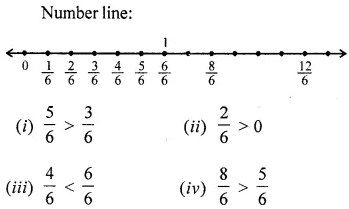Question 2.
Compare the given fractions and replace ‘….’ by an appropriate sign ”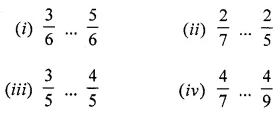Solution: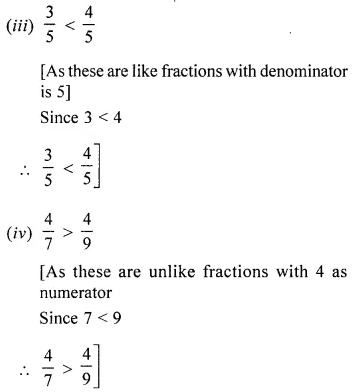Question 3.
Replace ‘…..’ by an appropriate sign ‘<, = or >’ between the given fractions:Solution: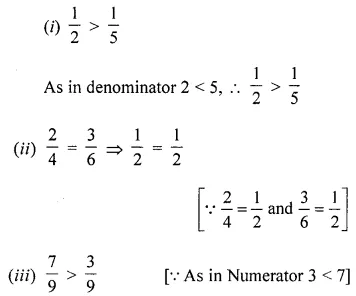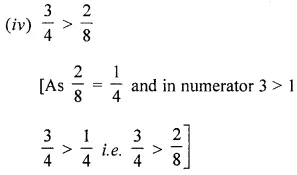Question 4.
Write the shaded portions as fractions. Arrange them in ascending order using appropriate sign between fractions:Solution: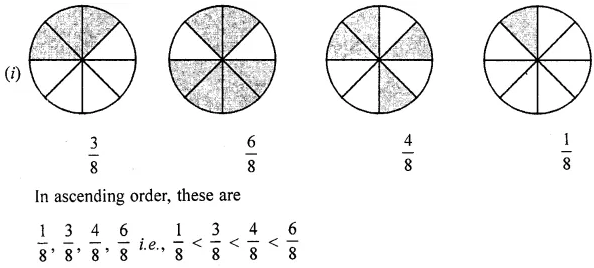Question 5.
Compare the following pairs of fractions:Solution: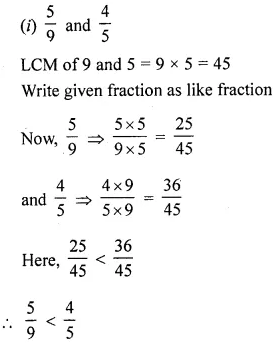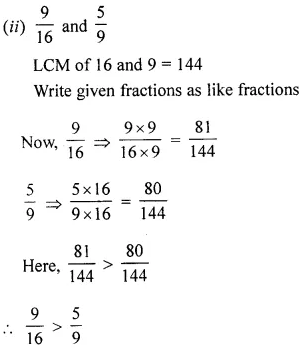Question 6.
Fill in the boxes by the symbol < or > to make the given statements true:Solution:Question 7.
Arrange the given fractions in descending order: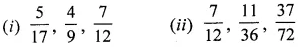Solution: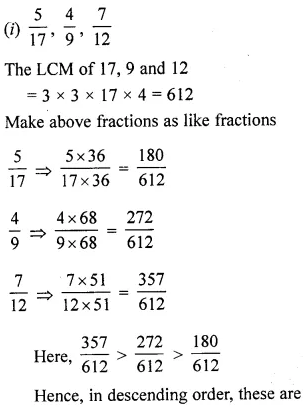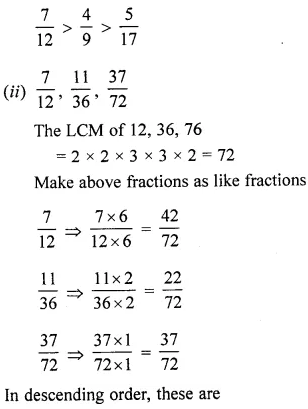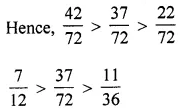Question 8.
Arrange the given fractions in the ascending order: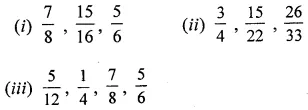Solution: